# M6-S3: Electromagnetic Induction### Magnetic Flux and Flux Density

• Magnetic flux is a measurement of the total number of magnetic field lines passing through a given area.
• Magnetic flux density (B) is a measurement of the density of magnetic field lines. It is also known as magnetic field strength
• Therefore, total magnetic flux in a given area is always equal to the flux density of that particular area multiplied by the area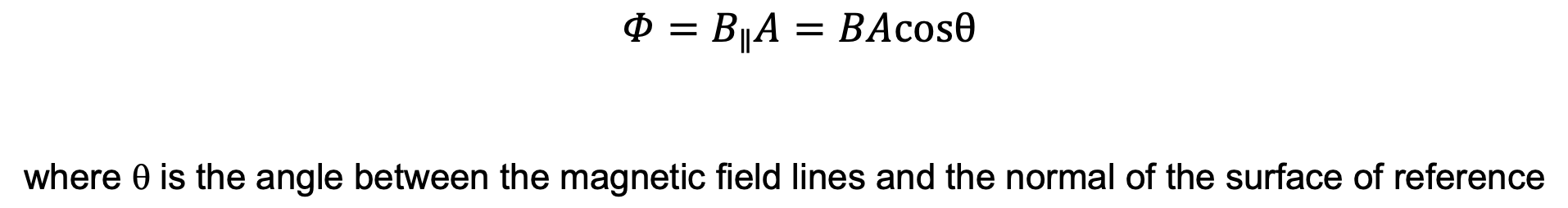• When the surface is parallel to the magnetic field lines, its normal is perpendicular to the magnetic field, thus total magnetic flux in that given area is zero.
• When the surface is perpendicular to the magnetic field lines, its normal is parallel to the magnetic field, thus total magnetic flux in that given area is maximum.
• The magnitude of magnetic flux is dependent on three things:
1. Magnetic flux density/magnetic field strength (B) in the given area
2. The orientation of the surface of reference in relation to the magnetic field
3. The area of the surface• Any changes to one or more of these three things will result in a change in magnetic flux passing through the given area. In other words, the area will experience a change in magnetic flux.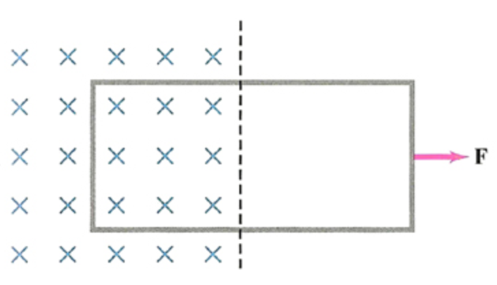• Changes in magnetic flux can also occur when the surface of area A is moved to a location with differing magnetic flux, either higher or lower.
• This including moving an object (e.g. conductor) in and out of a magnetic field.

 Quantity Magnetic Flux Magnetic Flux Density/Magnetic Field Strength (B) Area (A) Unit(s) Weber (Wb) Tesla (T) or Weber per metre squared (Wbm-2) Metre squared (m2) Scalar or Vector? Vector Vector Scalar

Currents in Straight Conductors and Solenoids

• The direction and orientation of magnetic field accompanying currents in straight conductors and solenoids can be both determined by the right-hand grip rule

 Straight Conductor Solenoid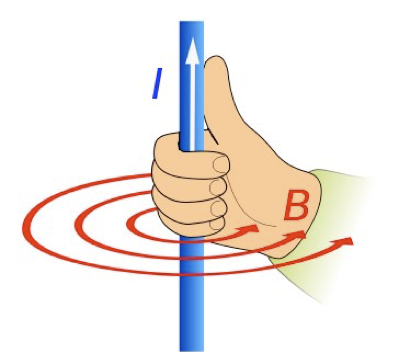·       Magnetic flux density decreases further away from the straight conductor ·       Constant magnetic flux density in the core of the solenoid. Flux density is reduced outside the solenoid   ·       Direction determined by right-hand grip rule. Thumb points in the direction from south to north pole. Currents flow in the direction of curled fingers.   ·       Effectively used to create electromagnets where the magnitude of the magnetic field B is proportional to the size of the current I#### Discovery of Electromagnetic Induction

• Michael Faraday wrapped wires around opposite ends of a soft iron ring, with one attached to a power source and another to a voltmeter.
• After the switch is closed, the current flow through the first wire causes a ‘wave’ of current to be created in the opposite wire. The induced current disappears after a short while.
• When a conductor is subjected to an external magnetic field, the free moving electrons (unaffected by potential difference) in the conductor experience a magnetic force in the same direction.• This movement of electrons creates current. However, after a short while, the movement of electrons will stop as the constant force causes them to end up in a particular extremity of the conductor e.g. one end of a straight conductive rod.
• Thus, a current is only induced when the magnetic field, experienced by the conductor is changing.

• Faraday’s Law of Induction: an emf (and accompanying current) is induced in a conductor when it experiences change in magnetic flux. In all conductors, free-moving electrons can form a current when an electromotive force (emf) is produced. This is commonly referred to as voltage which forces free-moving electrons to flow in a unified direction, leading to the formation of current.
• The magnitude of the emf is proportional to the rate of magnetic flux change. Faster the change, greater the induced current.• Energy transformation: induction of emf represents formation of electrical energy. This energy must have originated from somewhere due to law of conservation of energy. The origin of electrical energy depends on how the change in magnetic flux is produced
• When change in magnetic flux is changed by relative motion between a conductor and external magnetic field, kinetic energy à electrical energy. This transformation is evident when the relative motion slows down
• When the conductor has electrical resistance, the induced emf (electrical energy) may be partially converted into heat energy due to collisions between electrons and the lattice structure of the conductor. This is negligible when superconductors (zero resistance) are used.
• In his first experiment, the closing of the switch creates a current which in turn generates a magnetic field. Consequently, the second wire in the opposite end of the iron ring experiences a change in magnetic flux which leads to the induction of current. After a short period of time, the magnetic field generated by the current in the first wire becomes constant so the change in magnetic flux experienced by the second wire disappears, so does the induced current.

#### Relative Movement Between Magnetic Field and Conductors

• Relative movement between magnets and conductors causes relative motion of free moving electrons in the conductor. This produces a force which acts on the electrons in a uniform direction. Consequently, a current is induced.
• The relative movement can be achieved by:
1. Moving a magnet in the vicinity of a conductor
2. Moving a conductor within a non-uniform magnetic field
• In HSC physics, three types of conductors are analysed: straight conductors, solenoids and metal plates.

Straight Conductors

• When a straight conductor experiences changes in magnetic flux, a current is induced such that its accompanying magnetic field would oppose the change.
• The induced current would be in the direction such that the resultant magnetic force (Motor Effect) would restore the original position of the conductor. This is to restore the magnetic flux it was originally experiencing
• For example, if a straight conductor is moved out of a magnetic field (decrease in flux), the induced current would produce a magnetic force (direction can be determined by right-hand rule) in the direction towards the magnetic field.
• Vice versa, if a straight conductor is moved into a magnetic field (increase in flux), the result force from the induced current would be in the direction away from the magnetic field.

Solenoids

• Moving a permanent magnet in and out of a solenoid induces current.Observations:

• In a), the magnet is at rest so there is no deflection in the ammeter, so the needle of ammeter is at centre or zero position.
• In b), when the magnet is moved toward the coil, the needle of ammeter deflects in one direction.
• In c), when the magnet is held stationary at that position, the needle of ammeter returns back to zero position.
• In d), when the magnet is moved away from the coil, there is some deflection in the needle but in opposite direction.
• Notice that the direction of magnet’s motion determines the direction of current.
• Induced current generates its own magnetic field which in turn is orientated such that it opposes the external magnetic field. The generation of current in the coils shown above is inevitably accompanied by magnetic field. The orientation of this new magnetic field is determined by the right-hand rule (shown below)Why does the induced current oppose the external magnetic field?

• The resistance-like behaviour can be explained by law of conservation of energy. If the newly generated magnetic field instead reinforces the motion of the permanent magnet, its kinetic energy will increase due to an increase in velocity. For example, the newly created magnetic field will attract and repel the permanent magnet when it’s brought towards and away from the solenoid respectively. This increase in kinetic energy violates the law of conservation of energy
• Thus, when a conductor experiences a changing external magnetic field, a current is induced in a direction such that the associated magnetic field opposes the external magnetic field which produced the current in the first place. This is known as Lenz’s Law.

### What is Lenz's Law? The Law of Conservation of Energy in Lenz's Law

#### Lenz’s Law explains the resisting force observed when a conductor experiences change in magnetic flux

• Lenz’s law states

‘the direction of an induced current is such that the magnetic flux produced will oppose the change in flux which induced in the emf’

• In addition to the induced current observed in the solenoids above, the initial movement of the permanent magnet is opposed by the induced current’s own magnetic field

Explanation of Induced Current in Solenoids

• When the magnet is brought towards the solenoid, the solenoid experiences an increase in magnetic flux. As a result, a magnetic field is created to repel the incoming magnet. Therefore, its velocity is reduced.
• When the magnet is brought away from the solenoid, the solenoid experiences a decrease in magnetic flux. As a result, a magnetic field is created to attract the incoming magnet. Therefore, its velocity is reduced.
• When the magnet is fully inside the core of the solenoid, no changes in magnetic flux are experienced by the solenoid. Consequently, the solenoid does not need to oppose any external magnetic fields so, no current is induced.

Metal Plates & Metal Sheets

• The induced current in a metal plate as a result of Faraday and Lenz’s law is called eddy current
• Eddy currents are circular closed currents.
• Eddy currents are only generated in conductors of large surface area, usually metal plates, cylindrical pipes made of conducting material
• The direction of induced current (either clockwise or anti-clockwise) can be determined by the right-hand grip rule).
• Thumb points in the direction of the magnetic flux the metal plate desires to restore
• Curled fingers are in the direction of the eddy current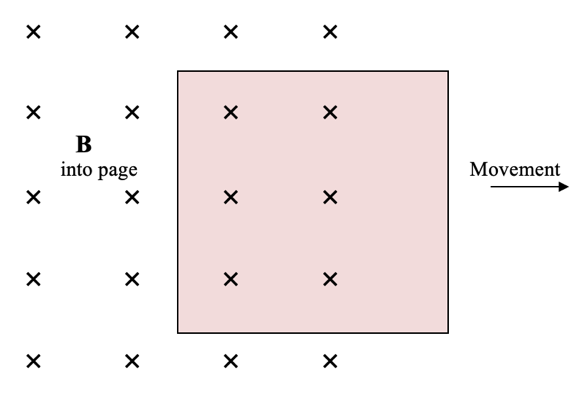• For example: in the diagram above, a square metal plate is moved out of the magnetic field going into the page. As a result, the metal plate experiences a decrease in magnetic flux. Specifically, a decrease of flux which are going into the page. So, it desires to increase the number of magnetic fluxes going into the page in order to oppose the change (Lenz’s Law).
• Thumb points into the page
• Thus, fingers are curled in a clockwise manner. This is the direction of the resultant eddy current.

#### Electromagnetic Induction Using Two Solenoids

• Since flowing current in a solenoid can produce a magnetic field that resembles a permanent magnet, two solenoids in close proximity can also display Faraday and Lenz’s law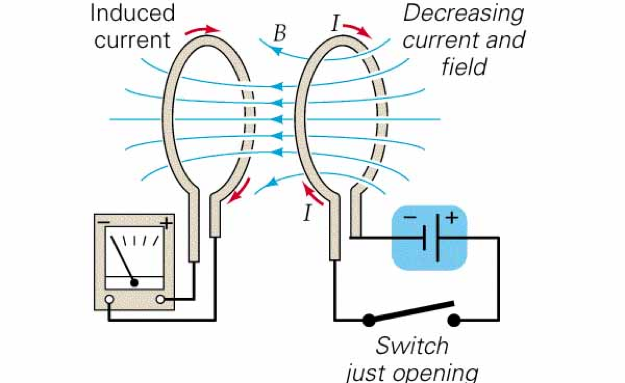• For example: in the diagram above, the loop of wire on the right is connected to a battery whilst the loop on the left is only connected to an ammeter
• Closing and opening the switch in the right loop cause the left loop to experience an increase and decrease in magnetic flux respectively.
• In both cases, by Faraday’s law, an induced current is detected by the ammeter.
• By Lenz’s law, the direction of current is different when the switch is closed compared with when it’s open. This is shown by the pointer being deflected in opposite direction in the
• Shortly after the switch has been closed or opened, the induced current in the left loop disappears. This is because, the magnetic flux experienced by the left loop becomes constant (no change).
• This way of transmitting electrical energy from one conductor to another is called flux linkage

Previous section: The Motor Effect and Forces Between a Pair of Straight Conductors

Next section: Applications of the Motor Effect: DC Motor